## Set

A set is a Finite or Infinite collection of objects. Older words for set include Aggregate and Class. Russell also uses the term Manifold to refer to a set. The study of sets and their properties is the object of Set Theory. Symbols used to operate on sets include(which denotes the Empty Set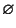),(which denotes the Power Set of a set),(which means and'' or Intersection), and(which means or'' or Union).

The Notation, whereandare arbitrary sets, is used to denote the set of Maps fromto. For example, an element ofwould be a Map from the Natural Numbers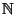to the set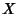. Call such a function, then,, etc., are elements of, so call them,, etc. This now looks like a Sequence of elements of, so sequences are really just functions fromto. This Notation is standard in mathematics and is frequently used in symbolic dynamics to denote sequence spaces.

Let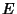,, and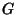be sets. Then operation on these sets using theandoperators is Commutative(1)(2)

Associative(3)(4)

and Distributive(5)(6)

More generally, we have the infinite distributive laws(7)(8)

whereruns through any Index Set. The proofs follow trivially from the definitions of union and intersection.

The table below gives symbols for some common sets in mathematics.

 Symbol Set-BallComplex Numbers,-Differentiable Functions-Disk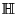QuaternionsIntegersNatural NumbersRational Numbers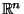Real Numbers in-D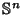-SphereIntegers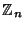integers (mod)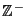Negative IntegersPositive IntegersNonnegative Integers

See also Aggregate, Analytic Set, Borel Set, C, Class (Set), Coanalytic Set, Definable Set, Derived Set, Double-Free Set, Extension, Ground Set, I, Intension, Intersection, Kinney's Set, Manifold, N, Perfect Set, Poset, Q, R, Set Difference, Set Theory, Triple-Free Set, Union, Venn Diagram, Well-Ordered Set, Z, Z-, Z+

References

Courant, R. and Robbins, H. The Algebra of Sets.'' Supplement to Ch. 2 in What is Mathematics?: An Elementary Approach to Ideas and Methods, 2nd ed. Oxford, England: Oxford University Press, pp. 108-116, 1996.